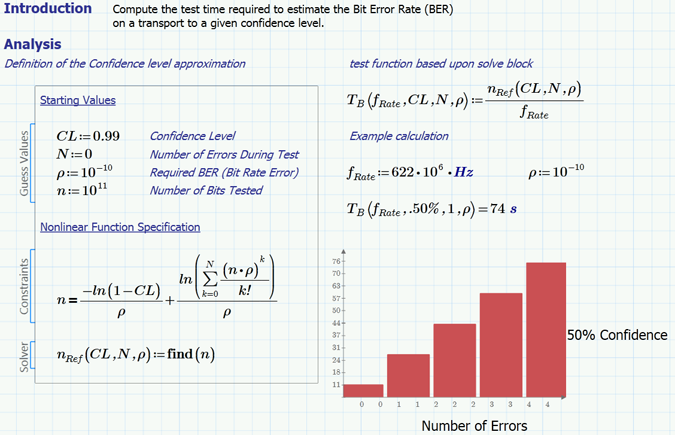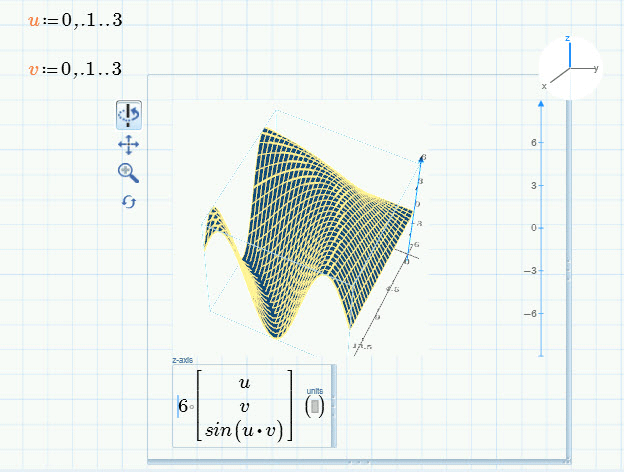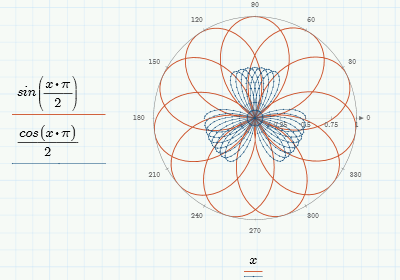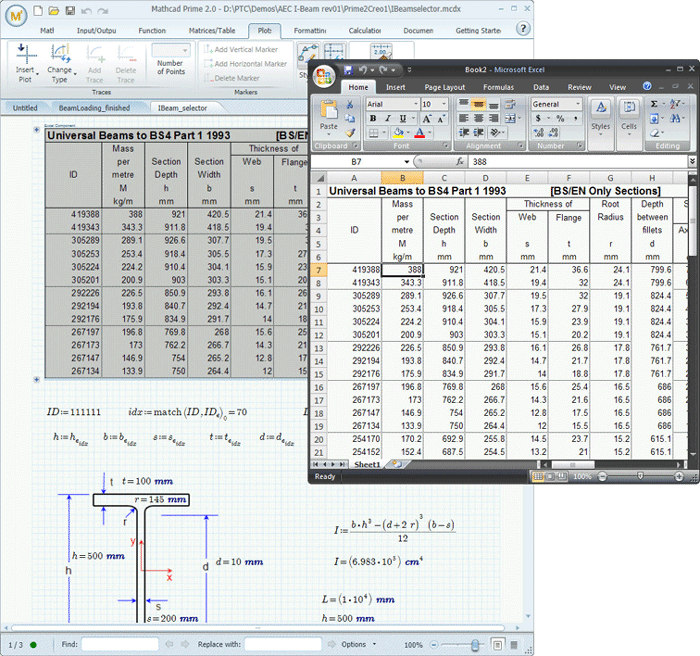# Engineering Calculations Software

## Mathcad Prime 2.0 is the Industry Standard Software for Engineering Calculations.

Calculations are the heart of all engineering designs. Engineers in industry and academia find themselves struggling with spreadsheets and engineering calculators to solve and document their engineering calculations.

Mathcad Prime 2.0 simplifies engineering calculations documentation through its document centric, what-you-see-is-what-you-get-approach. It is engineering calculations software that uses natural math layout and combines equations, text, and graphics, in a presentable format. With Mathcad engineers find it easy to solve, document, share and re-use complex calculations.

Mathcad brings the ease of an ‘engineering notebook’ to the powerful features of ‘dedicated engineering calculations software’.

• Document-centric environment
• Natural math layout
• No coding required, easy to use interface
• Full units support for reduced errors
• 2D & 3D PlotsClick on a image to view larger

Combine equations, graphs, images and text in one documentDirect Manipulation of 3D PlotsUse Natural Math Notation